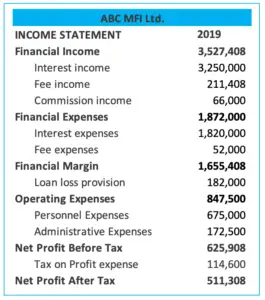# Financial Self Sufficiency

## Overview

Financial self-sufficiency is the ratio that is used for the evaluation of the company’s ability to generate revenue to cover its total costs while maintaining its equity value and other concession funds relative to the inflation and other cost of capital. This type of ratio is usually used in the microfinance institution.

The financial self-sufficiency ratio is similar to the operation self-sufficiency ratio where they both measure the company’s ability to generate revenue by comparing to the total cost, including operating expenses, financial cost, and loan loss provision expense. However, comparing to operational self-sufficiency, financial self-sufficiency is better in long term measurement.

This is due to financial self-sufficiency ratio takes into account the outside risk such as inflation. Hence, the imputed cost of capital is added as an additional cost in the calculation of this ratio.

## Financial Self Sufficiency Formula

The financial self-sufficiency ratio can be calculated using the formula below:• Operating income includes interest, fee, and commission income
• Operating expenses include personnel expenses and administrative expenses
• Financial cost includes interest and fee expenses on financing

It is useful to note that the imputed cost of capital is an invisible cost that does not incur directly like operating expenses or financial cost. And the inflation rate may be used in the calculation of the imputed cost of capital if there is no market rate of equity to use.

For example, if the inflation rate is 5% and the company’s USD 10 M performing assets come from funding which consists of 70% commercial loan and 30% equity, the imputed cost of capital equals USD 150K (10M x 30% x 5%) in amount.

## Financial Self Sufficiency Example

For example, we have the 2019 income statement of ABC MFI Ltd. as below.Assume that the imputed cost of capital equal to USD 223 K in amount after taking 5% inflation to the equity value (and assume no concession loan).

What is the financial self-sufficiency ratio of ABC MFI?

Solution:

With the financial information in the 2019 income statement above, we can get:

• Operating income = financial income = 3,527,408
• Operating expenses = 847,500
• Financial cost = 1,872,000
• Loan loss provision = 182,000
• Imputed cost of capital = 223,000

Hence, we can calculate the financial self-sufficiency ratio as below:

Financial self-sufficiency = 3,527,408 / (847,500 + 1,872,000 + 182,000 + 223,000) = 112.90%

So, the financial self-sufficiency ratio is 112.90 % which means that the company is doing well to earn sufficient operating revenues to cover its total cost as well as maintaining its value of equity relative to inflation.